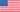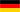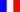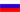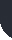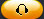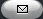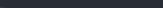Task Management Software for Team Collaboration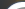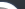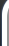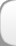HomeDownloadSupportPurchase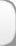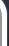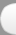Installation GuideStartUp GuideOnline Help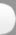# Total Cost and Cost Factor for All Project Phases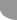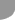Home  » Products  » VIP Task Manager Pro  » Tutorials  » Customization Guide  » Total Cost and Cost Factor for All Project Phases

Total Cost and Cost Factor for All Project Phases

Total Cost for All Project Phases is a project management KPI that shows the total cost spent on each phase of a project. Phase Cost Factor shows a proportion of certain project phase cost to the total project cost. One example of using this indicator measures can be construction project. Assume that a construction project consists of the following phases:

• Pre-project Planning
• Detail Design
• Procurement
• Construction
• Start-up/Commissioning

To calculate Phase Cost Factor you may use the formula below:

Phase Cost Factor = Actual Phase Cost / Actual Project Cost

Actual Phase Cost is the actual total cost spent on each phase of a project. For construction projects Actual Phase Cost can be calculated by the similar formula used to calculate Actual Cost of the entire project [Project Budget Factor]. You can create a separate task group per each project phase to calculate total Actual Phase Cost.

Actual Phase Cost = (Actual Work * Standard Rate) + (Actual Overtime Work * Overtime Rate) + Resource per Use Costs + Actual Cost of Materials + Other Expenses.

Actual Work, Actual Overtime Work, Actual Cost of Materials, Other Expenses are actual values calculated per project phase.

Actual Project Cost is the total amount spent from pre-project planning phase to Start-up/Commissioning project phase.

To calculate total cost spent on project phases please follow the steps below:

• Add custom field “Actual Phase Cost” with Code: cf_actual_phase_cost and the formula:

ToFloat(\$cf_actual_phase_work)*24 * \$cf_standard_rate + ToFloat(\$cf_actual_overtime_phase_work)*24 * \$cf_overtime_rate + \$cf_resource_per_use_cost + \$cf_phase_cost_of_materials + \$cf_other_expenses_per_phase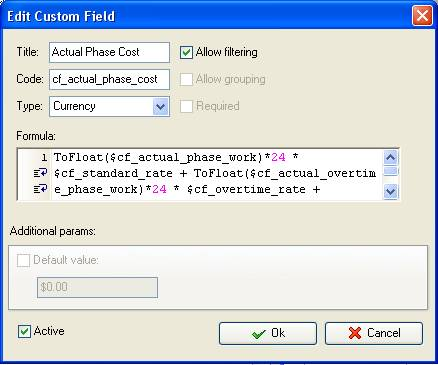• On Task Tree select the task group that corresponds to certain project phase and select “Custom fields options”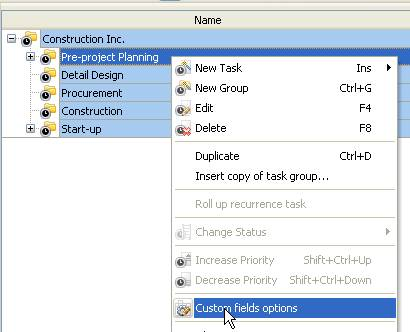• Set the following custom fields settings for the custom field: “Actual Phase Cost”
• In “Settings for” select “This task group”
• select “Enable”
• to display the total actual cost per each project phase select “Sum”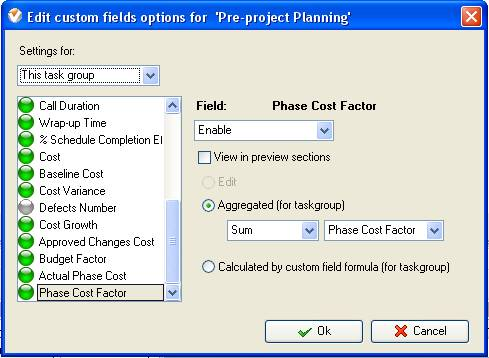Such settings should be configured for each project phase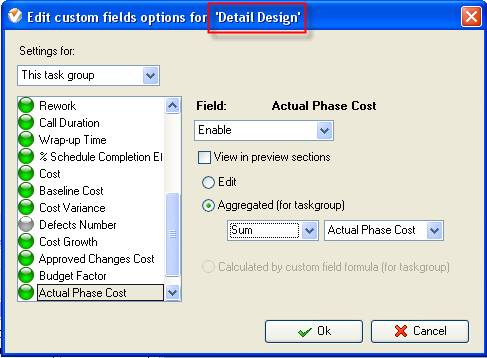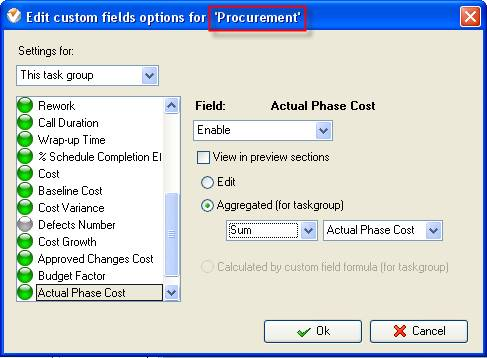Note: To enter the value of the custom field “Actual Phase Cost” phase you need to right click on task group that corresponds to particular phase, select “Custom fields options” and select “Edit”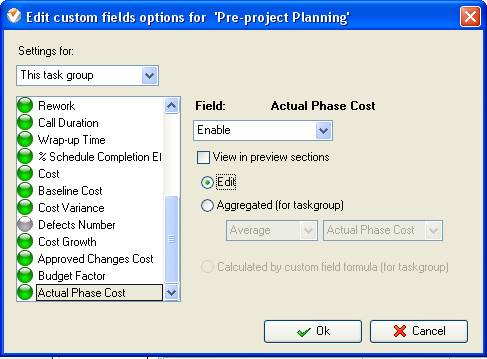To enter the actual cost per phase right click on task group and select “Edit”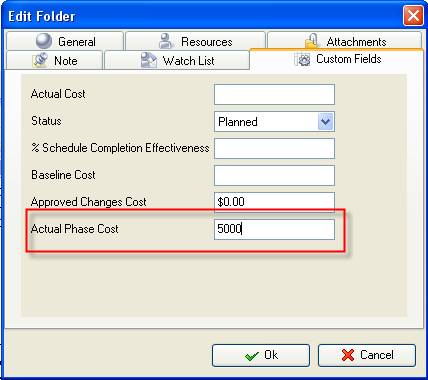• Select the root task group and set the following settings for the custom field “Actual Cost”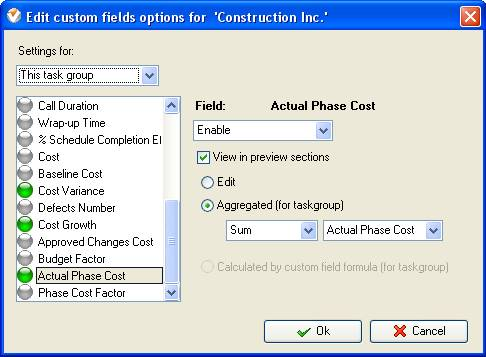To calculate Phase Cost Factor follow the step-by-step instructions below:

• Add custom field “Phase Cost Factor” with the formula:

\$cf_actual_phase_cost / X, where X is the value of the custom field “Actual Cost” displayed per root task group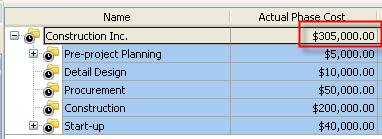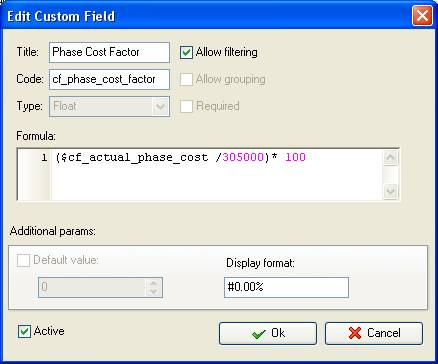• On Task Tree select the root task group and select “Custom fields options”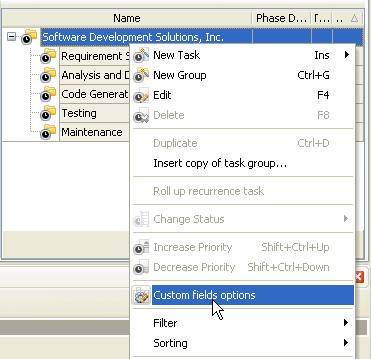• Set the following custom fields settings for the custom field “Phase Cost Factor”
• In “Settings for” select ”Subgroups&tasks
• select “Enable”
• select “Calculated by custom field formula (for task group)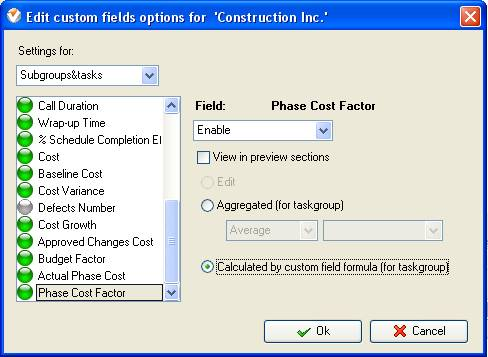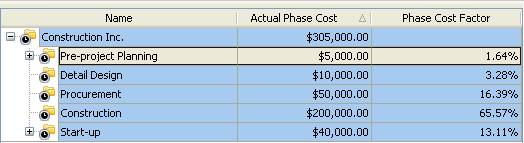Total Cost and Cost Factor for All Project Phases on Task Tree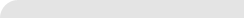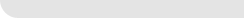» VIP Task Manager Pro VIP Task Manager Std Testimonials Turnkey Solution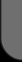ProductsSolutionsDownloadSupportPurchaseContactsForumVideoMy Account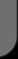Copyright © 2004 - 2023 Task Management Software